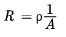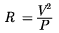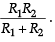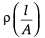Assertion & Reason Test: Electricity - 2

# Assertion & Reason Test: Electricity - 2

Test Description

## 15 Questions MCQ Test Online MCQ Tests for Class 10 | Assertion & Reason Test: Electricity - 2

Assertion & Reason Test: Electricity - 2 for Class 10 2022 is part of Online MCQ Tests for Class 10 preparation. The Assertion & Reason Test: Electricity - 2 questions and answers have been prepared according to the Class 10 exam syllabus.The Assertion & Reason Test: Electricity - 2 MCQs are made for Class 10 2022 Exam. Find important definitions, questions, notes, meanings, examples, exercises, MCQs and online tests for Assertion & Reason Test: Electricity - 2 below.
Solutions of Assertion & Reason Test: Electricity - 2 questions in English are available as part of our Online MCQ Tests for Class 10 for Class 10 & Assertion & Reason Test: Electricity - 2 solutions in Hindi for Online MCQ Tests for Class 10 course. Download more important topics, notes, lectures and mock test series for Class 10 Exam by signing up for free. Attempt Assertion & Reason Test: Electricity - 2 | 15 questions in 30 minutes | Mock test for Class 10 preparation | Free important questions MCQ to study Online MCQ Tests for Class 10 for Class 10 Exam | Download free PDF with solutions
 1 Crore+ students have signed up on EduRev. Have you?
Assertion & Reason Test: Electricity - 2 - Question 1

### Directions: In the following questions, a statement of assertion (A) is followed by a statement of reason (R). Mark the correct choice as:Assertion : When the length of a wire is doubled, then its resistance also gets doubled.Reason : The resistance of a wire is directly proportional to its length.

Detailed Solution for Assertion & Reason Test: Electricity - 2 - Question 1 The resistance of wire,i.e. R ∝ l Since, the resistance of a wire is directly proportional to its length, i.e. when the length of a wire is doubled/ halved then its resistance also gets doubled/halved.

Assertion & Reason Test: Electricity - 2 - Question 2

### Directions: In the following questions, a statement of assertion (A) is followed by a statement of reason (R). Mark the correct choice as:Assertion: The 200 W bulbs glow with more brightness than 100 W bulbs.Reason : A 100 W bulb has more resistance than a 200 W bulb.

Detailed Solution for Assertion & Reason Test: Electricity - 2 - Question 2 Resistance,R ∝ 1/p

i.e. Higher the wattage of a bulb, lesser is the resistance and so it will glow bright.

Assertion & Reason Test: Electricity - 2 - Question 3

### Directions: In the following questions, a statement of assertion (A) is followed by a statement of reason (R). Mark the correct choice as:Assertion : Positive charge inside the cell always goes from the positive terminal to the negative terminal.Reason : Positive charge inside the cell may go from negative terminal to the positive terminal.

Detailed Solution for Assertion & Reason Test: Electricity - 2 - Question 3 S.I. is true only when current is drawn from a cell.
Assertion & Reason Test: Electricity - 2 - Question 4

Directions: In the following questions, a statement of assertion (A) is followed by a statement of reason (R). Mark the correct choice as:

Assertion : A voltmeter and ammeter can be used together to measure resistance but not power.

Reason : Power is proportional to voltage and current.

Detailed Solution for Assertion & Reason Test: Electricity - 2 - Question 4 Resistance, R = V/I

Power, P = VI We can measure both resistance and power by measuring the V and I simultaneously in circuit. So option (e) is correct.

Assertion & Reason Test: Electricity - 2 - Question 5

Directions: In the following questions, a statement of assertion (A) is followed by a statement of reason (R). Mark the correct choice as:

Assertion : Electric appliances with metallic bodies have three connections, whereas an electric bulb has a two pin connection.

Reason : Three pin connections reduce heating of connecting wires.

Detailed Solution for Assertion & Reason Test: Electricity - 2 - Question 5 The metallic body of the electrical appliances is connected to the third pin which is connected to the earth. This is a safety precaution and avoids eventual electric shock. By doing this the extra charge flowing through the metallic body is passed to earth and avoids shocks. There is nothing such as reducing the heating of connecting wires by three pin connections.
Assertion & Reason Test: Electricity - 2 - Question 6

Directions: In the following questions, a statement of assertion (A) is followed by a statement of reason (R). Mark the correct choice as:

Assertion (A): The resistivity of the conductor increases with the increasing temperature.

Reason (R): The resistivity is the reciprocal of the conductivity.

Detailed Solution for Assertion & Reason Test: Electricity - 2 - Question 6 The resistivity of the conductors is directly proportional to temperature.
Assertion & Reason Test: Electricity - 2 - Question 7

Directions: In the following questions, a statement of assertion (A) is followed by a statement of reason (R). Mark the correct choice as:

Assertion (A): A conductor has + 3.2 × 10–19 C charge.

Reason (R): Conductor has gained 2 electrons.

Detailed Solution for Assertion & Reason Test: Electricity - 2 - Question 7 Conductor has positive charge, so it has lost two electrons.
Assertion & Reason Test: Electricity - 2 - Question 8

Directions: In the following questions, a statement of assertion (A) is followed by a statement of reason (R). Mark the correct choice as:

Assertion (A): Two resistance having value R each. Their equivalent resistance is R / 2

Reason (R): Given Resistances are connected in parallel.

Detailed Solution for Assertion & Reason Test: Electricity - 2 - Question 8 When two resistances R1 and R2 connected in parallel than their equivalent resistance will be R =Assertion & Reason Test: Electricity - 2 - Question 9

Directions: In the following questions, a statement of assertion (A) is followed by a statement of reason (R). Mark the correct choice as:

Assertion (A): Bending a wire does not affect electrical resistance.

Reason (R): Resistance of wire is proportional to resistivity of material.

Detailed Solution for Assertion & Reason Test: Electricity - 2 - Question 9 Resistance of wire

R =Where r is resistivity of material which does not depend on the geometry of wire. Since when wire is bent its resistivity, length and area of cross section do not change, therefore resistance of wire also remains same.

Assertion & Reason Test: Electricity - 2 - Question 10

Directions: In the following questions, a statement of assertion (A) is followed by a statement of reason (R). Mark the correct choice as:

Assertion (A): Silver is not used to make electric wires.

Reason (R): Silver is a bad conductor.

Detailed Solution for Assertion & Reason Test: Electricity - 2 - Question 10 Silver is a good conductor of electricity but it is not used to make electric wires because it is expensive.
Assertion & Reason Test: Electricity - 2 - Question 11

Directions: In the following questions, a statement of assertion (A) is followed by a statement of reason (R). Mark the correct choice as:

Assertion (A): The electric bulbs glow immediately when the switch is ON.

Reason (R): The drift velocity of electrons in a metallic wire is very high.

Detailed Solution for Assertion & Reason Test: Electricity - 2 - Question 11 In a conductor there are large numbers of free electrons. When we close the circuit, the electric field is established instantly with the speed of electromagnetic waves which causes electron drift at every portion of the circuit. Due to which the current is set up in the entire circuit instantly. The current which is set up does not wait for the electrons to flow from one end of the conductor to another end. Due to this, the bulb glows immediately when the switch is ON.
Assertion & Reason Test: Electricity - 2 - Question 12

Directions: In the following questions, a statement of assertion (A) is followed by a statement of reason (R). Mark the correct choice as:

Assertion (A): In a simple battery circuit the point of lowest potential is the positive terminal of the battery.

Reason (R): The current flows towards the point of the lower potential as it flows in such a circuit from the positive to the negative terminal.

Detailed Solution for Assertion & Reason Test: Electricity - 2 - Question 12 It is quite clear that in a battery circuit, the point of lowest potential is the negative terminal of the battery and the current flows from higher potential to lower potential.
Assertion & Reason Test: Electricity - 2 - Question 13

Directions: In the following questions, a statement of assertion (A) is followed by a statement of reason (R). Mark the correct choice as:

Assertion (A): Alloys are commonly used in electrical heating devices like electric iron and heater.

Reason (R): Resistivity of an alloy is generally higher than that of its constituent metals but the alloys have low melting points then their constituent metals.

Detailed Solution for Assertion & Reason Test: Electricity - 2 - Question 13 Alloys are held for electrical heating devices due to their light resistivity and high melting point compared to constituent metals.
Assertion & Reason Test: Electricity - 2 - Question 14

Directions: In the following questions, a statement of assertion (A) is followed by a statement of reason (R). Mark the correct choice as:

Assertion (A): Copper is used to make electric wires.

Reason (R): Copper has very low electrical resistance.

Detailed Solution for Assertion & Reason Test: Electricity - 2 - Question 14 A low electrical resistance of copper makes it a good electric conductor. So, it is used to make electric wires.
Assertion & Reason Test: Electricity - 2 - Question 15

Directions: In the following questions, a statement of assertion (A) is followed by a statement of reason (R). Mark the correct choice as:

Assertion (A): Electric appliances with a metallic body have three connections, whereas an electric bulb has a two pin connection.

Reason (R): Three pin connections reduce heating of connecting wires.

Detailed Solution for Assertion & Reason Test: Electricity - 2 - Question 15 The metallic body of an electrical appliance is connected to the third pin which is connected to the earth. This is a safety precaution and avoids eventual electric shock. By doing this the extra charge flowing through the metallic body is passed to earth and avoids shocks. There is nothing such as reducing the heating of connecting wires by three pin connections.

## Online MCQ Tests for Class 10

456 tests
 Use Code STAYHOME200 and get INR 200 additional OFF Use Coupon Code
Information about Assertion & Reason Test: Electricity - 2 Page
In this test you can find the Exam questions for Assertion & Reason Test: Electricity - 2 solved & explained in the simplest way possible. Besides giving Questions and answers for Assertion & Reason Test: Electricity - 2, EduRev gives you an ample number of Online tests for practice

456 tests